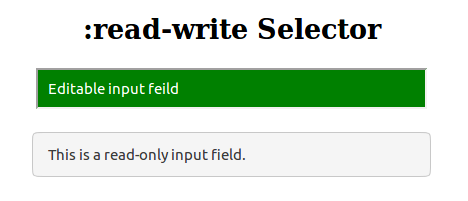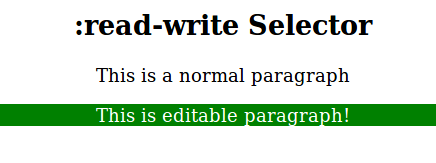Related Articles
• Last Updated : 05 Nov, 2019

The :read-write selector is used to select an element (such as an input text) that is editable by the user. The elements with no readonly and disabled attribute are defined as readable and writable.

Syntax:

```:read-write {
// CSS Property
}```

Example 1:

 ` ` `<``html``> ` `    ``<``head``> ` `        ``<``title``>:read-write Selector ` `        ``<``style``> ` `            ``input { ` `                ``min-width: 25em; ` `                ``padding: 10px; ` `            ``} ` `             `  `            ``/* CSS property for Firefox only */ ` `            ``input:-moz-read-write {  ` `                ``background: green; ` `                ``color: white; ` `            ``} ` `             `  `            ``input:read-write {  ` `                ``background: green; ` `                ``color: white; ` `            ``} ` `        `` ` `    `` ` `    ``<``body` `style` `= ``"text-align:center"``> ` `         `  `        ``<``h2``> ` `            ``:read-write Selector ` `        `` ` `        ``<``input` `type` `= ``"text"` `value` `=  ` `        ``"Editable input field"``><``br``><``br``> ` `         `  `        ``<``input` `type` `= ``"text"` `value` `=  ` `        ``"This is a read-only input field."` `readonly> ` `    `` ` `                     `

Output:Example 2:

 ` ` `<``html``> ` `    ``<``head``> ` `        ``<``title``>:read-write Selector ` `        ``<``style``> ` `            ``p:-moz-read-write {  ` `                ``background: green; ` `            ``} ` `            ``p:read-write {  ` `                ``background: green;  ` `            ``}  ` `            ``p[contenteditable = "true"] {  ` `                ``color: white; ` `            ``} ` `        `` ` `    `` ` `     `  `    ``<``body` `style` `= ``"text-align:center"``> ` `        ``<``h2``> ` `            ``:read-write Selector ` `         `` ` `          `  `        ``<``p``> ` `            ``This is a normal paragraph ` `        `` ` `        ``<``p` `contenteditable` `= ``"true"``> ` `            ``This is editable paragraph! ` `        `` ` `    `` ` `                     `

Output:Supported Browsers: The browser supported by :read-write Selector are listed below:

• Apple Safari 9.0